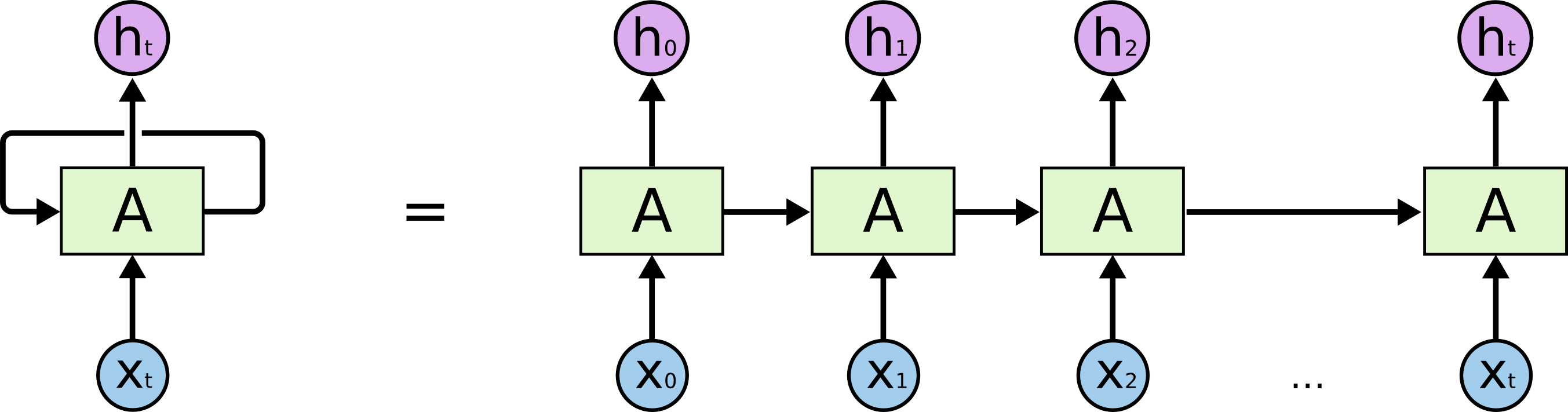# The difference between Dense and TimeDistributedDense of Keras

I am still confused about the difference between Dense and TimeDistributedDense of Keras even though there are already some similar questions asked here and here. People are discussing a lot but no common-agreed conclusions.

And even though, here, @fchollet stated that:

TimeDistributedDense applies a same Dense (fully-connected) operation to every timestep of a 3D tensor.

I still need detailed illustration about what exactly the difference between them.

Let's say you have time-series data with $N$ rows and $700$ columns which you want to feed to a SimpleRNN(200, return_sequence=True) layer in Keras. Before you feed that to the RNN, you need to reshape the previous data to a 3D tensor. So it becomes a $N \times 700 \times 1$.The image is taken from https://colah.github.io/posts/2015-08-Understanding-LSTMs



In RNN, your columns (the "700 columns") is the timesteps of RNN. Your data is processed from $t=1 \ to \ 700$. After feeding the data to the RNN, now it have 700 outputs which are $h_1$ to $h_{700}$, not $h_1$ to $h_{200}$. Remember that now the shape of your data is $N \times 700 \times 200$ which is samples (the rows) x timesteps (the columns) x channels.

And then, when you apply a TimeDistributedDense, you're applying a Dense layer on each timestep, which means you're applying a Dense layer on each $h_1$, $h_2$,...,$h_t$ respectively. Which means: actually you're applying the fully-connected operation on each of its channels (the "200" one) respectively, from $h_1$ to $h_{700}$. The 1st "$1 \times 1 \times 200$" until the 700th "$1 \times 1 \times 200$".

Why are we doing this? Because you don't want to flatten the RNN output.

Why not flattening the RNN output? Because you want to keep each timestep values separate.

Why keep each timestep values separate? Because:

• you're only want to interacting the values between its own timestep
• you don't want to have a random interaction between different timesteps and channels.
• And then, when you apply a TimeDistributedDense, you're applying a Dense layer on each timestep --> This mean each timestep share the Dense layer's weight? With the Dense layer doesn't it only apply to the last timestep? Jan 21, 2018 at 9:19
• Why isn't TimeDistributedDense not used in the Keras example at blog.keras.io/… ? Mar 7, 2018 at 11:48
• Because TimeDistributedDense is already deprecated. Since Keras 2.0, Dense can handle >2-dimensional tensor well Jan 24, 2019 at 9:23
• It is explained perfectly, one more clarification I need to ask: the whole point of running a TimeDisterbutedDense is to properly reduce the number of the channels for each timestep to one, am I correct? Feb 22, 2022 at 14:20